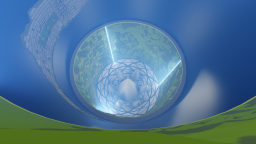Rama physically-based lighting

In our previous renderings of Rama the shading was inspired by the physical lighting equations, but was manually tweaked to get "beautiful" results (in particular for the atmosphere). Here we want to know how Rama would really look like, using the "exact" physical lighting equations, without any manual tweak, and based on the results obtained in the physics of Rama. Also, unlike for our previous renderings, which were using a high resolution geometric model but were not real-time, we would like here a real-time rendering with a low resolution geometric model (having a high resolution model rendered in real-time is a long term goal which is not adressed here). In summary our goals are:

• a real-time rendering of a low-resolution Rama model,
• with a strong emphasis on physically-based ligthing.

Our general strategy to acheive these goals is roughly the following:

• we first generate a static and low resolution geometric model of Rama, with proper texture coordinates referring to a texture atlas,
• we then pre-compute a lightmap using the previous texture parameterization and the "exact" physical lighting equations,
• finally we render the low resolution geometric model in real-time, using the pre-computed lightmap.
In practice however, things are a bit more complex because of the atmosphere and the cylindrical sea. These yield participating media and specular effects which are view-dependent and thus cannot be pre-computed in the lightmap. Instead, we pre-compute them, partially, in other data structures, as explained below. In the following we briefly summarize the main results from the physics of Rama which are important for the lighting, and then explain how we (pre-)compute the direct and indirect lighting in Rama. We conclude with the results obtained using this method.

## 1 Physics of Rama

If we assume that Rama should provide an environement as close as possible to the one on Earth (in terms of grativy, lighting, temperature, pressure, etc), then we showed in our study of the physics of Rama, that:
• the 6 linear light sources can not have any shape. For instance a cylindrical shape would yield very large temperatures on the ground near the light sources. We found that a flat shape, with each point of the light source emitting light equally in all directions, was a good choice to get uniform ground temperatures.
• the 6 linear light sources must provide a total power of $6.7\,MW.m^{-1}$ in order the get an illuminance of about $35.000\,lux$ at the ground level (on Earth, the illuminance when the Sun is at the zenith, with a clear sky, is about $100.000\,lux$). This result is based on the hypothesis that the light sources emit only in the visible range, without producing any heat. The corresponding irradiance at the ground level is then $157\,W.m^{-2}$.
• the flat linear light sources give an almost uniform temperature $T_s=15°C$ at the ground level. This in turn gives an almost uniform temperature $T_a=T_s$ in the atmosphere, and finally determines a unique profile for the pressure and for the scattering coefficients. The result is $k_s(r)=k_s(r_s) \exp\left(-\frac{\omega^2}{R_sT_a}\frac{r_s^2-r^2}{2}\right)\,m^{-1}$ where $k_s(r_s)$ is the scattering coefficient value at the ground level (cf our notations). It is supposed to have the same value as on Earth, i.e. $5.8\,10^{-6}\,m^{-1}$ for $\lambda=680\,nm$, $13.5\,10^{-6}\,m^{-1}$ for $\lambda=550\,nm$, and $33.1\,10^{-6}\,m^{-1}$ for $\lambda=440\,nm$.
• because of the uniform temperature in the atmosphere, there can be no clouds. And since clouds contribute a lot to the Earth's average albedo ($\alpha_e=0.3$), the average albedo in Rama must be smaller. The above results assumed an average albedo $\alpha=0.15$.

## 2 Rama Model

In order to generate our low resolution Rama model we combine several closed 3D meshes with Constructive Solid Geometry (using the Carve library):
• An axially symmetric mesh for the general shape of the inner surface of Rama.
• Seven cones for the horns in the south pole.
• Six rectangular cuboids for the light source "trenches".
• Six terrain meshes generated from "high resolution" height maps (10 meters per pixel), mapped to a cylinder, and downsampled to about 35000 triangles each with the Surface Simplification Using Quadric Error Metrics algorithm.
• A 3D mesh for the Manhattan island generated from a 2D contour polygon of the island and 3D prisms for the blocks of buildings (we then triangulate the ground part with the triangle library and map the final result to a cylinder).

The result is a watertight mesh with normals and a texture parameterization (designed manually, as opposed to being generated by an automatic packing algorithm). We then associate basic materials to this geometric model. We use purely diffuse materials everywhere, except for the cylindrical sea (where we combine a purely diffuse component with a specular component). In order to get a realistic physically-based lighting, we need to use plausible albedo values for each material. We chose the following values:

 Sample RGB albedo Avg. albedo Material (0.15, 0.15, 0.15) 0.15 Road asphalt. (0.2, 0.2, 0.2) 0.2 North pole, south pole, horns, Manhattan buildings. (0.13, 0.18, 0.1) 0.13 Forests. (0.2, 0.24, 0.06) 0.16 "Grass" around the six terrains. (0.16, 0.22, 0.06) 0.14 Crops. (0.17, 0.24, 0.06) 0.15 Crops. (0.24, 0.25, 0.09) 0.19 Crops. (0.3, 0.29, 0.13) 0.24 Crops. (0.18, 0.29, 0.08) 0.18 Crops. (0.31, 0.36, 0.06) 0.24 Crops. (0.24, 0.4, 0.08) 0.24 Crops. (0.12, 0.24, 0.16) 0.17 Rivers. (0.0026, 0.020, 0.072) 0.03 Cylindrical sea (diffuse part).

based on typical albedo values from Wikipedia, the Encyclopedia of Earth and NASA. For the cylindrical sea albedo we use the values from Rendering Natural Waters for a "coastal ocean" with "type I" water: the albedo is then $0.33b_b/a$, using the notations from the paper, which evaluates to the above values. The average RGB albedo for the whole Rama model is then equal to (0.10, 0.12, 0.09), or 0.1 when averaged across wavelengths.

## 3 Direct Lighting

Given the above physical model, we can now compute the direct ligthing in Rama, i.e. the light that goes directly from a light source L to the eye E, or which is reflected only once in between, either on a diffuse surface D, on a specular surface S, or by a volume V (e.g. the atmosphere). We detail each case in the following sections.

## 3 Indirect Lighting

The direct light from the linear light sources that bounces off from diffuse and specular surfaces, or which is scattered by the atmosphere, acts a seconday (area and volumetric) light source. Since the average albedo in our Rama model is about 0.1, this secondary light source contributes for about 10% of the direct light, which cannot be neglected (on the other hand, the contribution of indirect light is 1% after 3 bounces, 0.1% after 4 bounces, etc, so we can neglect these contributions).

Thus our goal here is to compute the indirect light from the light source L to the eye E after exactly two bounces on a diffuse surface D, on a specular surface S, or by a volume V (e.g. the atmosphere). This gives 9 possible types of path, such as LDDE, LVDE, etc. Here we neglect the paths of type LSDE (caustics on surfaces), LSVE (caustics in volumes) and LSSE (specular reflection of a specular reflection) for simplicity (also we believe that their contribution is small, because the cylindrical sea represents only 20% of the total surface). This leaves 6 other types of paths, that we examine in the following sections.

## 4 Results

The final result and the contributions from each type of light path is shown below, for a single light source: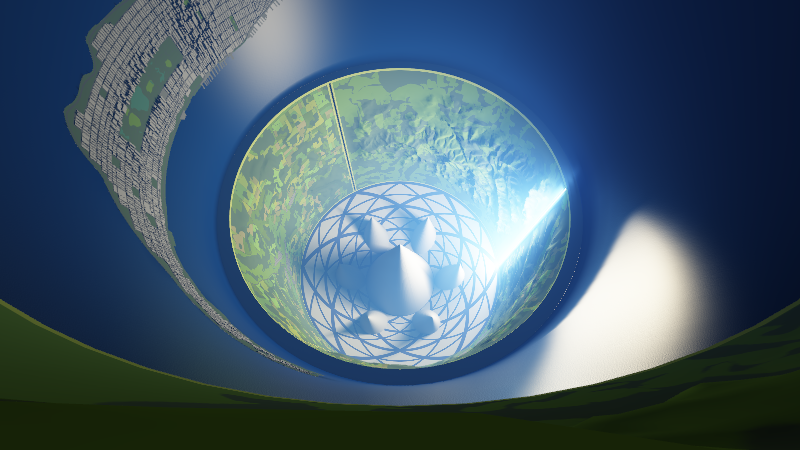Figure 1: LE paths LDE paths LSE paths LVE path LDDE and LVDE paths LDSE and LVSE paths LDVE and LVVE paths All paths.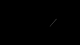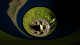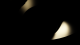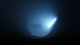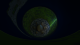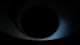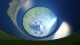and for the 6 linear light sources (using a smaller exposure):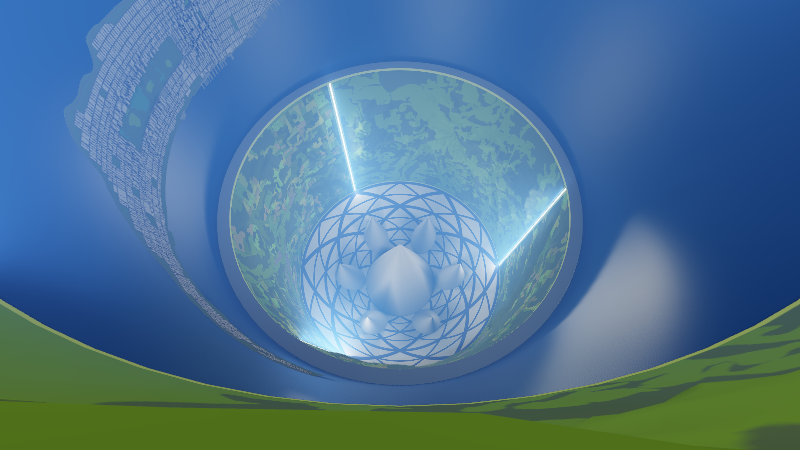Figure 2: LE paths LDE paths LSE paths LVE path LDDE and LVDE paths LDSE and LVSE paths LDVE and LVVE paths All paths.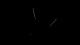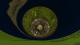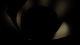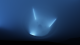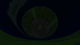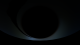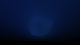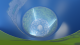## 5 Demo

Our low resolution Rama model is available as a 3D mesh in OBJ file format. It has about 136.000 vertices and 273.000 triangles. The texture contains the physical albedo values (multiplied by 255 to be stored in 8 bits).

We also provide a real-time demo where the user can navigate in Rama with a helicopter-like vehicle, and view each light path contribution separately or combined with others, for a single or six linear source(s), and with or without ground textures (see the screenshot below). The demo provides a precompiled binary for Windows, as well as the full source code and a simple Makefile.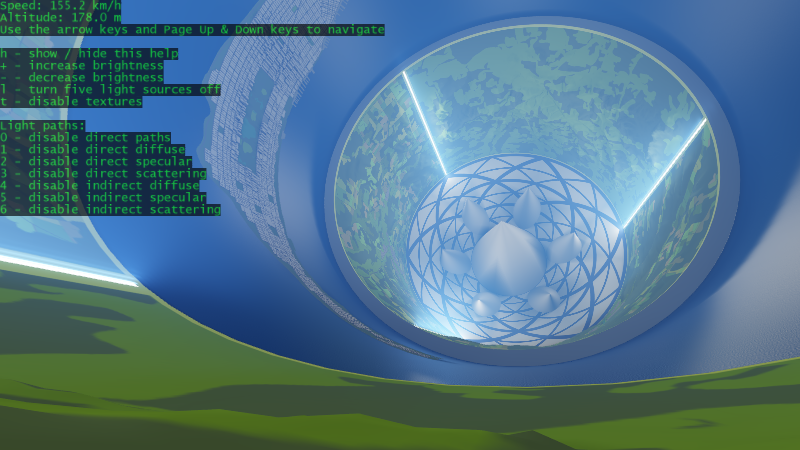Figure 3: Screenshot of the real-time Rama demo.